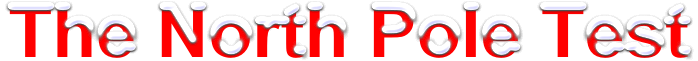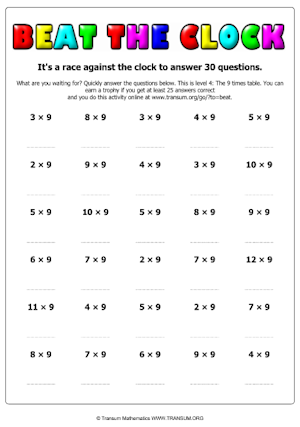#It is very cold at the North Pole, so cold that your brain could stop working.

Show that your brain is working well right now by writing out the 99 times table.

How far can you get in five minutes?North Pole
99 miles

Try this activity again when you are feeling cold. Can you get as far in five minutes?## A Chilling Mathematics Lesson Starter Of The Day

Share

Topics: Starter | Tables | Xmas

You can find many more ChristMaths activities at:

Transum.org/ChristMaths/• Primary 6 & Mrs Thom, Netherlee PS
•
• In five minutes, our primary 6 class managed to get the first 16 stations correct. We felt that it was tricky but a lot of fun. Some were frustrated that if they got one answer wrong then every other answer was also wrong.

We really enjoyed today's challenge!
• Matthew, 3D, Craigslea State School
•
• I got up to the 29th station in 5 minutes.
It was fun!
• Katee Wheeler, ------
•
• This starter has some potential and is very helpful and interesting for developing Mathematical skills.
• Debbie, Guernsey
•
• Very absorbing activity! One of my Y5s managed up to 49 x 44 in 5 minutes.

[Transum: If are reading this comment and wondering why it refers to the 44 times table while you have been presented with another times table it is because a different number appears every time this page is loaded.]
• Transum,
•
• Yes, the cold can really affect your brain in a negative way. Perhaps a chilly day where you live won’t make a big difference but for really cold environments there have been studies which have found that mental changes (reflecting a cooling brain) are the first sign of hypothermia. The tell-tale signs include becoming withdrawn, slower movements, poor coordination, hallucinations, slurred speech, poor memory and eventually loss of consciousness.

How did you use this starter? Can you suggest how teachers could present or develop this resource? Do you have any comments? It is always useful to receive feedback and helps make this free resource even more useful for Maths teachers anywhere in the world.

If you don't have the time to provide feedback we'd really appreciate it if you could give this page a score! We are constantly improving and adding to these starters so it would be really helpful to know which ones are most useful. Simply click on a button below:

Excellent, I would like to see more like this
Good, achieved the results I required
Satisfactory
Didn't really capture the interest of the students
Not for me! I wouldn't use this type of activity.

This starter has scored a mean of 3.0 out of 5 based on 187 votes.

Previous Day | This starter is for 17 December | Next Day

1 x 99 = 99
2 x 99 = 198
3 x 99 = 297
4 x 99 = 396
5 x 99 = 495
6 x 99 = 594
7 x 99 = 693
8 x 99 = 792
9 x 99 = 891
10 x 99 = 990
11 x 99 = 1089
12 x 99 = 1188
13 x 99 = 1287
14 x 99 = 1386
15 x 99 = 1485
16 x 99 = 1584
17 x 99 = 1683
18 x 99 = 1782
19 x 99 = 1881
20 x 99 = 1980
21 x 99 = 2079
22 x 99 = 2178
23 x 99 = 2277
24 x 99 = 2376
25 x 99 = 2475
26 x 99 = 2574
27 x 99 = 2673
28 x 99 = 2772
29 x 99 = 2871
30 x 99 = 2970
31 x 99 = 3069
32 x 99 = 3168
33 x 99 = 3267
34 x 99 = 3366
35 x 99 = 3465
36 x 99 = 3564
37 x 99 = 3663
38 x 99 = 3762
39 x 99 = 3861
40 x 99 = 3960
41 x 99 = 4059
42 x 99 = 4158
43 x 99 = 4257
44 x 99 = 4356
45 x 99 = 4455
46 x 99 = 4554
47 x 99 = 4653
48 x 99 = 4752
49 x 99 = 4851
50 x 99 = 4950
51 x 99 = 5049
52 x 99 = 5148
53 x 99 = 5247
54 x 99 = 5346
55 x 99 = 5445
56 x 99 = 5544
57 x 99 = 5643
58 x 99 = 5742
59 x 99 = 5841
60 x 99 = 5940
61 x 99 = 6039
62 x 99 = 6138
63 x 99 = 6237
64 x 99 = 6336
65 x 99 = 6435
66 x 99 = 6534
67 x 99 = 6633
68 x 99 = 6732
69 x 99 = 6831
70 x 99 = 6930
71 x 99 = 7029
72 x 99 = 7128
73 x 99 = 7227
74 x 99 = 7326
75 x 99 = 7425
76 x 99 = 7524
77 x 99 = 7623
78 x 99 = 7722
79 x 99 = 7821
80 x 99 = 7920
81 x 99 = 8019
82 x 99 = 8118
83 x 99 = 8217
84 x 99 = 8316
85 x 99 = 8415
86 x 99 = 8514
87 x 99 = 8613
88 x 99 = 8712
89 x 99 = 8811
90 x 99 = 8910
91 x 99 = 9009
92 x 99 = 9108
93 x 99 = 9207
94 x 99 = 9306
95 x 99 = 9405
96 x 99 = 9504
97 x 99 = 9603
98 x 99 = 9702
99 x 99 = 9801
100 x 99 = 9900

Note to teacher: Doing this activity once with a class helps students develop strategies. It is only when they do this activity a second time that they will have the opportunity to practise those strategies. That is when the learning is consolidated. Click the button above to regenerate another version of this starter from random numbers.

There are a printable worksheets for the more common timestables.Your access to the majority of the Transum resources continues to be free but you can help support the continued growth of the website by doing your Amazon shopping using the links on this page. Below is an Amazon search box and some items I have chosen and recommend to get you started. As an Amazon Associate I earn a small amount from qualifying purchases which helps pay for the upkeep of this website.Teacher, do your students have access to computers?Do they have iPads or Laptops in Lessons? Whether your students each have a TabletPC, a Surface or a Mac, this activity lends itself to eLearning (Engaged Learning).Transum.org/go/?Start=December17

Here is the URL which will take them to a game that will help them practise the 99 times table.

Transum.org/go/TD/?T=99

Here is the URL which will take them to the most popular multiplication tables app.

Transum.org/go/?to=tablesmasterFor All: